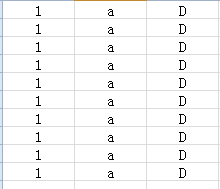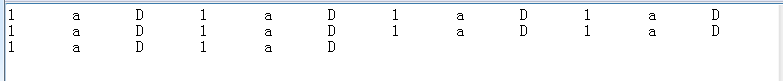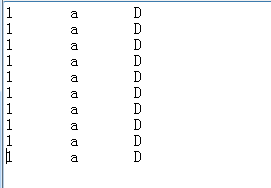excel宏定义问题，输出前三列到txt 5C

Sub st()
Dim rg As Object
Dim Strtemp
Dim rc As Long
rc = Application.Max(Range("A" & Rows.Count).End(xlUp).Row, Range("B" & Rows.Count).End(xlUp).Row)

Strtemp = ""
For Each rg In Range("A1:B" & rc)
If rg.Row <= rc Then
If rg.Column = 1 Then
Strtemp = Strtemp & rg.Value & vbTab
Else
Strtemp = Strtemp & rg.Value & vbCrLf
End If

``````End If
``````

Next
Open ThisWorkbook.Path & "\AB.txt" For Output As #1
Print #1, Strtemp
Close #1

End Sub

3个回答

``````Sub st()
Dim rg As Object
Dim Strtemp
Dim rc As Long
rc = Application.Max(Range("A" & Rows.Count).End(xlUp).Row, Range("B" & Rows.Count).End(xlUp).Row, Range("C" & Rows.Count).End(xlUp).Row)

Strtemp = ""
For Each rg In Range("A1:C" & rc)
If rg.Row <= rc Then
If rg.Column <> rc Then
Strtemp = Strtemp & rg.Value & vbTab
Else
Strtemp = Strtemp & rg.Value & vbCrLf
End If
End If
Next
Open ThisWorkbook.Path & "\AB.txt" For Output As #1
Print #1, Strtemp
Close #1

End Sub
``````3 年多之前 回复

excel 内容如图：O(∩_∩)O谢谢！

If rg.Column <> rc Then

If rg.Column <> 3 Then

``````Sub st()
Dim rg As Object
Dim Strtemp
Dim rc As Long
rc = Application.Max(Range("A" & Rows.Count).End(xlUp).Row, Range("B" & Rows.Count).End(xlUp).Row, Range("C" & Rows.Count).End(xlUp).Row)

Strtemp = ""
For Each rg In Range("A1:C" & rc)
If rg.Row <= rc Then
If rg.Column <> 3 Then
Strtemp = Strtemp & rg.Value & vbTab
Else
Strtemp = Strtemp & rg.Value & vbCrLf
End If
End If
Next
Open ThisWorkbook.Path & "\AB.txt" For Output As #1
Print #1, Strtemp
Close #1

End Sub
``````